Name:    Math 9 Quiz 8.1 and 8.2 Circle Geometry

True/False
Indicate whether the statement is true or false.

1.

The diameter of a circle is also a chord: TRUE or FALSE?

2.

The radius of a circle is also a chord:  TRUE or FALSE?

Multiple Choice
Identify the choice that best completes the statement or answers the question.

3.

Which of the following set of numbers is a pythagorean triple?
I) 6, 8, 10           II) 7, 24, 25           III) 8, 17, 19     IV) 12, 35, 37
 a. I and II only c. I, II, and IV only b. I, III, and IV only d. All of them

4.

What is the length of AB in the diagram accurate to 1 decimal place::a. 6.7 c. 13.4 b. 10.1 d. 16.4

5.

What is the length of AB in the diagram: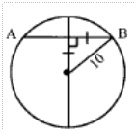a. 7.07 c. 21.21 b. 10.606 d. 14.14

6.

What is the length of AB in the diagram:a. 13.42 c. 12 b. 15 d. 12.55

7.

What is the length of the radius:a. 3.46 c. 9.73 b. 3.67 d. 6.93

8.

What is the length of AB?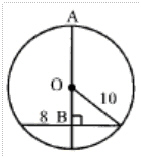a. 16 c. 10 b. 17.25 d. 8

9.

Given that the length of CD is 2 and ED is 8, then what is the length of AB?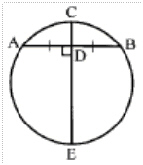a. 8 c. 10.4 b. 8.7 d. 12

10.

What is the length of “x” in the diagram?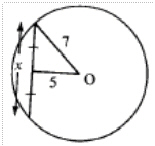a. 4.99 c. 7.39 b. 9.8 d. 12.4

11.

What is hte length of “x” in the diagram?a. 8 c. 6 b. 6.5 d. 7.2

12.

What is the length of BY?a. 14 c. 17.1 b. 21 d. 16

13.

What is the length of “z”?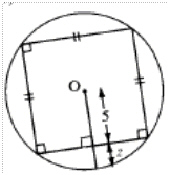a. 2.07 c. 4.12 b. 3.13 d. 1.16